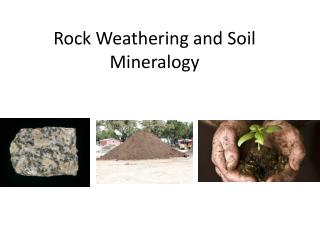Download PresentationRock Weathering and Soil Mineralogy

# Rock Weathering and Soil Mineralogy

Download Presentation## Rock Weathering and Soil Mineralogy

- - - - - - - - - - - - - - - - - - - - - - - - - - - E N D - - - - - - - - - - - - - - - - - - - - - - - - - - -
##### Presentation Transcript

1. Rock Weathering and Soil Mineralogy

2. Physical Weathering……

3. Weathering A . Chemical Weathering • Water is critical (and heat serves as a catalyst) • Complex Process (involves several reactions) • Biological Activity (helps accelerate weathering processes)

4. Complex Process • Solution • Hydrolysis • Oxidation • Hydration • Chelation • Dialysis • Chemical Reconstitution

5. The negative log of hydrogen ion content

6. Why does it matter?

7. Why does it matter? Most Mobile Least Mobile Ca2+ > Na+ > Mg2+ > K+ >Si4+>Fe3+> Al3+

8. Molar BA Ratio

9. Molar BA Ratio Uncle Festus Cuzzin Cletus

10. Molar BA Ratio Moles of Na2O + K2O + CaO + MgO Moles of Al2O3

11. Cation Exchange Capacity (CEC): The amount of exchangeable cations that a given weight of soil is capable of holding

12. CEC is measured in units of Cmol/kg, which replaces milliequivilents (meq)/100 g.

13. CEC is measured in units of Cmol/kg, which replaces milliequivilents (meq)/100 g. Wt. of 1 meq. = mole weight (g) charge of ion * 1000 Ex: the weight of 1 meq. of calcium is: 1 meq. Of Ca2+= 40.08 g = 0.02 g (2) * 1000

14. Ex: the weight of 1 meq. of calcium is: 1 meq. Of Ca2+= 40.08 g = 0.02 g (2) * 1000 So….the CEC of a soil containing 1.0316 g of exchangeable Ca2+per 100 g of soil is: 1.0316 g (wt. of exchangeable Ca2+) 0.02 (wt. of 1 meq. of Ca2+)

15. Ex: the weight of 1 meq. of calcium is: 1 meq. Of Ca2+= 40.08 g = 0.02 g (2) * 1000 So….the CEC of a soil containing 1.0316 g of exchangeable Ca2+per 100 g of soil is: 1.0316 g (wt. of exchangeable Ca2+) 0.02 (wt. of 1 meq. of Ca2+) = 51.58 meq. =51.58 meq. Per 100 g, or 51.58 Cmol+/kg

16. C. CEC Capacities

17. Base Saturation

18. Base Saturation: the percentage of cation exchange capacity of a soil occupied by basic cations. Why important? Plant growth Plant protection

19. Ultisols Alfisols Mollisol